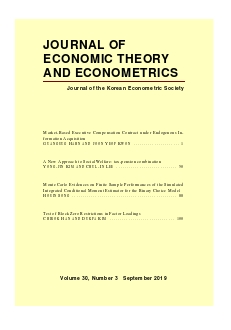상세검색
최근 검색어 전체 삭제
다국어입력
즐겨찾기0KCI등재 학술저널

# Examining the Commonality in Liquidity and Volatility Risk

• 등재여부 : KCI등재
• 2019.12
• 13 - 41 (29 pages)
• 2

We estimate latent factor models of liquidity and volatility. Common liquidity and volatility factors are extracted using multiple liquidity and volatility measures. Additionally, a latent factor is extracted by aggregating across both liquidity and volatility resulting in what we call the common uncertainty factor. The underlying uncertainty factor is correlated with the individual and the common liquidity and volatility factors as well as returns. We find that the underlying uncertainty risk factor is significantly priced in the cross section of expected returns, while the risks associated solely with liquidity and volatility are not suggesting that liquidity risk and volatility risk in stocks are related and should not be considered separately.

1. INTRODUCTION

2. DATA

3. CORRELATION ANALYSIS

4. JOINT PRICING OF LIQUIDITY AND VOLATILITY RISK IN THE CROSS-SECTION

5. CONCLUSION